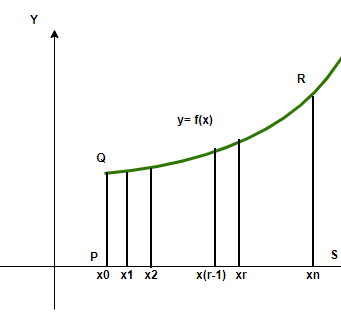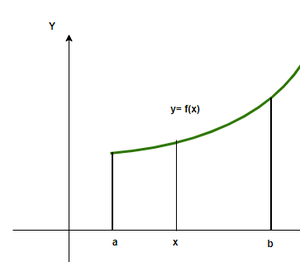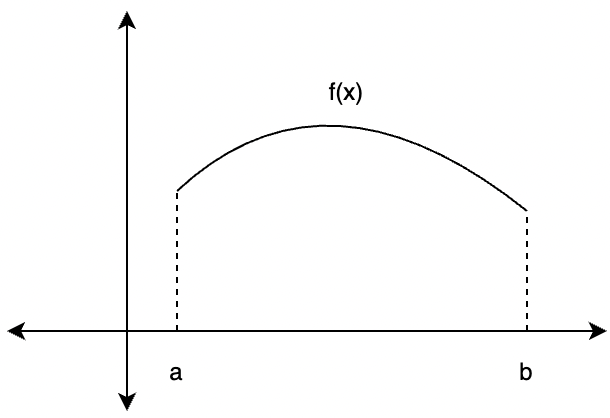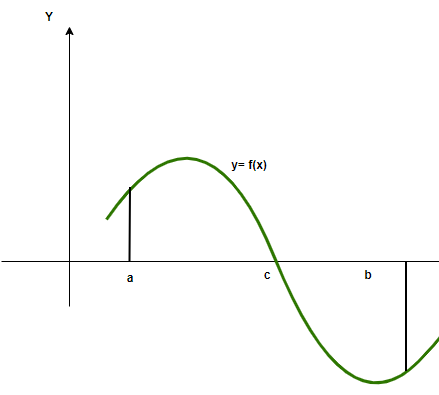Related Articles

# Computing Definite Integrals

• Last Updated : 24 Jun, 2021

Integrals are a very important part of the calculus. They allow us to calculate the anti-derivatives, that is given a function’s derivative, integrals give the function as output. Other important applications of integrals include calculating the area under the curve, the volume enclosed by a surface, etc. While the previous application mostly involves indefinite integrals, the latter one requires the boundaries to be well-defined and the integral is calculated in between those boundaries only. Such integrals are called definite integrals.

### Definite Integrals

Definite integrals are mostly used to calculate the areas and the volumes enclosed by the curves. Usually, the area is calculated using well-defined formulas for rectangles, circles, and squares, etc. In real life the shapes are not that simple, thus for calculating the areas for any arbitrary shape we use the definite integrals. For a function f(x) defined on the interval [a, b], the definite integral between these limits is given by,Here a is called the lower limit of the function and b is called the higher limit of the function.

Given a function f(x) that is continuous on the interval [a, b] we divide the interval into n subintervals of equal width,and from each interval choose a point. Then the definite integral of f(x) from a to b is,The above figure explains the limit definition, as we increase the number of rectangles under the curve the approximated area becomes closer and closer to the actual area under the figure.

### Fundamental Theorem of Calculus

The area of the region bounded by the curve f(x) between the ordinates x = a and x = b and the x-axis is given by. Let’s say x is any point between the limits, thenrepresents the area of the region from a to x. In other words, the area of this shaded region from “a” up until the value x is called the area function. It is given by,Based on this definition, two fundamental theorems of calculus are defined.

First Fundamental Theorem of Calculus

Let f(x) be a continuous function on the closed interval [a, b] and let A(x) be the area function.

Then, A'(x) = f(x), for all x ∈ [a, b].

Second Fundamental Theorem of Calculus

Let f(x) be a continuous function on the closed interval [a, b] and let F(x) be the anti-derivatives of the function f(x). Then,### Area under the Curve

The area under the curve is given by the definite integrals. We know that the area is always a positive quantity, but while using the definite integrals, sometimes the area that comes out is negative. For example, consider the two functions below, one lies completely above the x-axis, the other one has some part that lies below the x-axis. In such cases, the two areas might cancel out each other.In this case, the area is given by,For this case, the negative and positive areas must be calculated separately and only the magnitude of their areas must be added.Definite Integrals of Rational Function

For calculating the definite integrals for such functions, these functions are broken down using algebraic manipulation.

Question: Calculate the value of the following integralSolution:For calculating the definite integrals for such functions, we use the reverse power rule.

Question: Calculate the value of the following integralSolution:Using the reversed power rule,Definite Integrals of Trigonometric Functions

For calculating the definite integrals for such functions, we use the reverse power rule.

Question: Calculate the value of the following integralSolution:Definite Integrals of Natural Logarithmic Function

For calculating the definite integrals for such functions, we use the following identity.Question: Calculate the value of the following integralSolution:Using the formula mentioned above,Let’s look at some sample problems

### Sample Problems

Question 1: Calculate the value of the following integralSolution:Question 2: Calculate the value of the following integralSolution:Question 3: Calculate the value of the following integralSolution:Using the reversed power ruleQuestion 4: Calculate the value of the following integralSolution:Question 5: Calculate the value of the following integralSolution:Using the formula mentioned above,Attention reader! Don’t stop learning now. Join the First-Step-to-DSA Course for Class 9 to 12 students , specifically designed to introduce data structures and algorithms to the class 9 to 12 students

My Personal Notes arrow_drop_up